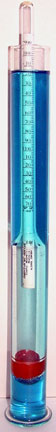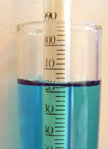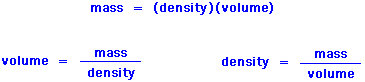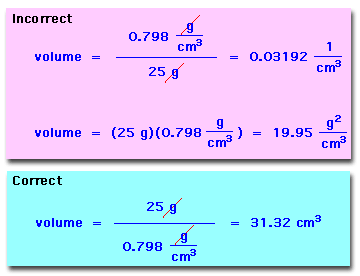MSDSTopics Free Sites FAQ's Regulations Glossary Software Suppliers Books Forum Poll Fun stuff Quiz Store Understand your MSDS with the MS-Demystifier Search ALL our MSDS info

Density, Specific gravityDon't let density measurements weigh you down. Get your laboratory balances and scales from Safety Emporium.

### Definition

Density and specific gravity have very similar, but not quite identical, definitions.

• Density is the amount of something per unit volume. Most typically, one expresses the mass per unit volume for a solid or liquid. For example, 5.2 g/cm3. For gases or dusts we might express this as g/m3.
• Specific gravity is a ratio of the mass of a material to the mass of an equal volume of water at 4 oC (39 oF). Because specific gravity is a ratio, it is a unitless quantity. For example, the specific gravity of water at 4 oC is 1.0 while its density is 1.0 g/cm3.
• Relative density is essentially the same as specific gravity, however the temperature used for the water (or even another material) is not necessarily 4 oC. For this reason, a relative density measurement will include the temperatures used for both materials. For example, "relative density 15/0: 0.87" indicates that the density of the material was determined at 15 oC and it is being divided by the density of water at 0 oC. The temperatures may also be indicated as a superscript (material) and subscript (water) after the numeric value.The specific gravity of a liquid can be determined with a hydrometer, a hollow, sealed, calibrated glass tube. The depth to which the hydrometer sinks is inversely proportional to the specific gravity of the liquid.

In the closeup below, we see that the specific gravity of the blue liquid is 1.016.Specific gravities can be determined in many other ways including pycnometry (weighing a known volume), and x-ray crystallography

At 4 oC, the density of water is 1.0 g/cm3. Therefore, density and specific gravity have the same numeric value at this temperature.

As we change temperature the volumes of different materials change in different ways. Most, but not all, materials expand (occupying a greater volume) as temperature is raised, meaning that their densities decrease. As the change in volume of water and other materials are not generally equal, the density and specific gravity may no longer be identical as temperature is changed.

For most solid materials, the volume change going from 4 oC to room temperature (20 oC, typically) is not very large. Therefore, we often use the terms density and specific gravity interchangeably as these values will not differ by more than one or two percent in most cases. For liquids, the volume changes with temperature can be more substantial. For gases, pressure in a closed system or volume in an open system increase or decrease proportionally with temperature as dictated by the Ideal Gas Law.

The density or specific gravity can be handy if we know the mass of material that was shipped to us but we need to know the volume...or vice-versa. To interconvert mass, volume and density, we can use any of the following equations which are simply rearranged forms of each other:.

A common error in such conversions is to divide instead of multiplying or vice-versa. This doesn't require memorizing the three different versions of the equation. All we have to do is write out the units and make sure that they cancel properly.

For example, if we have 25 g of a material with a density of 0.798 g/cm3, what size container will we require?.

Notice that the first two methods give units that make no physical sense and are certainly not volume units. If we always write our units, we will not make a mistake in density/mass/volume calculations.

For those of you who prefer the "black box" approach, the following Javascript calculator is helpful. If you put in two of the three values, the calculator will display the third one. The calculator assumes that your density units are g/cm3.

 Density Conversion Calculator(Enter two known values to calculate the third) gramscm3g/cm3 gramscm3g/cm3

Miscellaneous notes:

1. The Baumé scale is an obsolete alternative to using specific gravity.
2. Another outmoded (and annoying) density convention is the term mg%. This ambiguous term is equivalent to a mg/dL and is usually used in reference to blood serum levels (of cholesterol, bilirubin, toxins etc.)

Densities of some common materials are balsa wood (0.16 g/cm3), pine wood (0.5 g/cm3), gasoline (0.75 g/cm3), soybean oil (0.92 g/cm3), aluminum (2.7 g/cm3)), granite (2.75 g/cm3), lead (11.3 g/cm3), mercury (13.5 g/cm3) and gold (19.3 g/cm3). The densest material on Earth (not counting subatomic particles) is iridium metal (22.65 g/cm3).

### SDS Relevance

If known, the relative density of a material will be reported in Section 9 (physical and chemical properties) of the Safety Data Sheet.

As described above, density can be used to help determine volume or mass. Another use for specific gravity is to tell us if the material will sink or float in water or other liquid (assuming that it does not dissolve, of course). For example, a rock with a density of 4.3 g/cm3 will sink in water (density = 1.0 g/cm3), but a piece of plastic with a density of 0.8 g/cm3 will float in water.

When reading an SDS, remember the following:

1. If we have two equal volumes of a substance, the one with the larger density will be heavier.
2. If we have two equal masses of a substance, the one with the larger density will occupy less space (volume).Promote a culture of safety with Safety Scoreboards like this one from Safety Emporium.